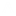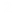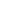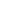```
1 {-
2
3 Pearl 6: Making a century
4
5 Problem:
6 Given the digits 1..9, list all the ways the operations + and x can be inserted into
7 the sequence so as to make the total sum 100.
8 For example:
9 100 = 12 + 34 + 5x6 +7 + 8 + 9
10 100 = 1 + 2x3 + 4 + 5 + 67 + 8 + 9
11
12 No parthneses, normal order of operations.
13
14 Solution:
15 This is a brute-force problem, but the goal of the pearl is to establish some
16 general formulation of 'Brute-Force' search, and improve on it with 'little'
17 assumptions.
18
19 Two main things for brute-force methods:
20 1. Generating the 'values' while generating the 'candidates', can produce savings (Especially
21 if generations of candidates can be 'sequential').
22 2. Reduce 'good' critertia to 'ok', it can help trim the generation of all sequences.
23
24 -}
25
26 -- You CAN skip all the general formulation description and go to ======
27
28 -- General formulation:
29 -- candidates    :: Data -> [Candidate]
30 -- value        :: Candidate -> Value
31 -- good         :: Value -> Bool
32
33 -- Brute force search over all candidates
34 -- solutions     :: Data -> [Candidate]
35 -- solutions    =  filter (good.value).candidates
36
37 -- Now, using a few assumptions we can modify thigns:
38
39 -- Assumption 1: This will allow us to fuse operations.
40 --
41 -- Data is a list of values, [Datum], and candidates takes the form
42 -- candidates :: [Datum] -> [Candidate]
43 -- candidates =  foldr extend []
44 -- where
45 -- extend :: Datum -> [Candidate] -> [Candidate]
46 --
47 --
48 -- Assumption 2: This will allow us to extend search only as needed.
49 --
50 --      2.a There is a predicate 'ok', such that every good value is necesserialy 'ok'
51 --      2.b candidates with 'ok' values are the extension of candidates with 'ok' value.
52 --
53 -- Assumption 3: This will save us computing 'value' of candidate
54 --
55 --   map value.extend x = modify x.map value
56 --  The values of an extended set can be computed from the values of which the extension was
57 --  built from.
58 --
59 --  We then introduce a few operations on 'fork', 'cross', and relates these
60 -- to zip and unzip.
61 --   fork (f,g) x          =  (f x, g x)
62 --   cross (f,g) (x,y)     = (f x, g y)
63 --
64 -- and we get:
65
66 -- solutions     ::     Data -> [Candidate]
67 -- solutions     =     map fst.filter (good.snd).foldr expand []
68
69 -- expand x     =     filter (ok.snd).zip.cross (extend x, modify x).unzip
70
71 module P6 where
72
73 import Data.List (intercalate)
74
75 -- ================================================
76
77
78
79 -- So all the above was general design-framework.
80 -- now, to the specific problem (creating 100)
81
82 -- Expression     = sum of terms
83 -- Term         = products of factors
84 -- Factor         = sequence of digits
85
86                             -- Candidate solution are expressions built from + and x
87                             -- Remember: No parnthesis etc, and simple prioity.
88 type Expression    = [Term]    -- Each expression is the sum of Terms
89 type Term         = [Factor]    -- Each term is the product of factors
90 type Factor     = [Digit]    -- Each factor is list of digits
91 type Digit         = Int
92
93
94 -- Just computing the value:
95 valExpr        ::    Expression -> Int
96 valExpr         =    sum.map valTerm
97 valTerm      = product.map valFact
98 valFact      = foldl (\n d -> 10*n+d)  0
99
100
101 good     ::     Int -> Bool
102 good v     =     (v==100)
103
104 ok        ::    Int -> Bool
105 ok v     =     (v<=100)
106
107 -- Creating all possible expressions
108 -- They mention there is a function 'partitions', but never spell it out.
109 -- so here it is, even though we will NOT use it!
110 partitions :: [Digit] -> [[[Digit]]]
111 partitions [] = [[]]
112 partitions (x:xs) = [[x]:p | p <- partitions xs]                -- x is a prt of it's own
113                  ++ [(x:ys):yss | (ys:yss) <- partitions xs]    -- x is with the next ones
114
115 -- A difffernet way
116 expressions xs = foldr extend [] xs
117
118 extend                     ::    Digit -> [Expression] -> [Expression]
119 extend x []             =    [[[[x]]]]
120 extend x es             =    concatMap (glue x) es
121
122 -- glue: Each new digit can be added in one of 3-different ways to the expression:
123 -- as a digit; as factor (multiplication); or as a term ==> 3 options.
124 -- BTW, this shows the number of possible expressions is 3^(n-1)
125 glue                     ::    Digit -> Expression -> [Expression]
126 glue x ((xs:xss):xsss)    =     [((x:xs):xss):xsss,       -- digit
127                              ([x]:xs:xss):xsss,        -- factor
128                              [[x]]:(xs:xss):xsss]    -- term
129
130
131 -- Main
132
133 main = do
134     -- putStr "Testing partitions: "
135     -- print \$ partitions [1..4]
136
137     putStrLn "Brute force : "
138     let res = filter (good.valExpr) \$ expressions [1..9]
139     --print res
140     prettyPrint res
141
142
143 -- pretty
144 prettyPrint es         =     putStr \$ unlines \$ map printExpression es
145
146 printExpression     ::  Expression -> String
147 printExpression e     =     "100 = " ++
148                         ( intercalate  " + " \$ map printTerm e)
149
150 printTerm t         =      intercalate  "*" \$ map printFactor t
151 printFactor f         =      concat \$ map show f
```